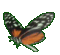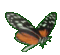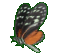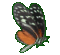Math Help - Rates & Ordered Pairs - Game Tips:

- For this game, it's not the size of one co-ordinate that counts, its the ratio that counts.
- The individual numbers in the ratio 4/2 are larger than the numbers used in the ratio 3/1,
but the ratio itself 4/2 = 2 is actually smaller than 3/1 = 3.

- Example 1: The rate = slope from a point P(2,6) to a point Q(3,10)
is calculated as a ratio = slope = (change in y)divided by(change in x) = (10-6)/(3-2) = (4)/(1)= 4.
The rate = 4  represents the speed with which y changes, relative to changes in x.

- Example 2: The rate of change in y=3x and its ordered pairs (1,3), (2,6), (3,9), (4,12),...
can be found using any two of its ordered pairs.
Using (1,3) and (2,6) the rate of change = (6-3)/(2-1) = 3/1 = 3
or using (2,6) and (3,9) the rate of change = (9-6)/(3-2) = 3/1 = 3
or using (1,3) and (4,12) the rate of change = (12-3)/(4-1) = 9/3 = 3 .

- The rate of change in a relation such as y=mx and its ordered pairs (x,y)
can be measured by the ratio = (change in y)/(change in x) = m = slope.
Generally, the idea is that the average rate = (y2-y1)/(x2-x1) = m = slope.

- Your Game Score is reduced by the number of butterfly hits.

- To slow the game speed repeat tap/click on the word Slider.
- To increase the game speed repeat tap/click on the word Math.
- Speed can also be adjusted with a keyboard's - and + keys.

- Refresh/Reload the web page to restart the game.